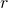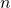# Determinantal and permanental representations of companion sequences associated to the r-Fibonacci sequence

Hacène Belbachir and Ihab-Eddine Djellas
Notes on Number Theory and Discrete Mathematics
Print ISSN 1310–5132, Online ISSN 2367–8275
Volume 28, 2022, Number 1, Pages 64—74
DOI: 10.7546/nntdm.2022.28.1.64-74

## Details

### Authors and affiliations

Hacène BelbachirUSTHB, Faculty of Mathematics, RECITS Laboratory
Po. Box 32, El Alia, 16111, Bab Ezzouar, Algiers, Algeria

Ihab-Eddine DjellasUSTHB, Faculty of Mathematics, RECITS Laboratory
Po. Box 32, El Alia, 16111, Bab Ezzouar, Algiers, Algeria

### Abstract

Recently companion sequences of-Fibonacci sequence were defined. The aim of this paper is to give some determinantal and permanental representations of these sequences via Hessenberg matrices. Several representations of classical sequences and polynomials are established. We conclude by using our representations to giveconsecutive terms of companion sequences simultaneously.

### Keywords

• Generalized bivariate r-Fibonacci polynomials
• Generalized bivariate r-Lucas polynomials
• Companion sequences
• Determinant
• Permanent
• Hessenberg matrix

• 15A15
• 11B39
• 11B37

### References

1. Abbad, S., Belbachir, H., & Benzaghou, B. (2019). Companion sequences associated to the r-Fibonacci sequence: Algebraic and combinatorial properties. Turkish Journal of Mathematics, 43(3), 1095–1114.
2. Cahill, N. D., D’Errico, J. R., Narayan, D. A., & Narayan, J. Y. (2002). Fibonacci
determinants. The College Mathematics Journal, 33(3), 221–225.
3. Chen, Y.-H., & Yu, C.-Y. (2011). A new algorithm for computing the inverse and the determinant of a Hessenberg matrix. Applied Mathematics and Computation, 218(8), 4433–4436.
4. Henrici, P. (1974). Applied and Computational Complex Analysis (Vol. 1). Wiley.
5. Ikebe, Y. (1979). On inverses of Hessenberg matrices. Linear Algebra and Its Applications, 24, 93–97.
6. Kaygisiz, K., & Sahin, A. (2012). Generalized bivariate Lucas p-polynomials and
Hessenberg Matrices. Journal of Integer Sequences, 15, Article 12.3.4.
7. Minc, H. (1964). Permanents of (0, 1)-circulants. Canadian Mathematical Bulletin, 7(2), 253–263.
8. Minc, H. (1984). Permanents (Vol. 6). Cambridge University Press.
9. Öcal, A. A., Tuglu, N., & Altinişik, E. (2005). On the representation of k-generalized Fibonacci and Lucas numbers. Applied Mathematics and Computation, 170(1), 584–596.
10. Tuglu, N., Kocer, E. G., & Stakhov, A. (2011). Bivariate Fibonacci like p-polynomials. Applied Mathematics and Computation, 217(24), 10239–10246.